Function Repository Resource:

# TriangularGridGraph

Create a triangular grid graph

Contributed by: Eric Weisstein (with contributions by Jibiana Jakpor)
 ResourceFunction["TriangularGridGraph"][n] creates a triangular grid graph from a triangular lattice of order n+1.

## Details

ResourceFunction["TriangularGridGraph"] takes the same options as Graph.

## Examples

### Basic Examples (2)

A triangular grid graph:

 In:=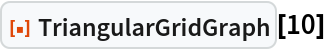Out=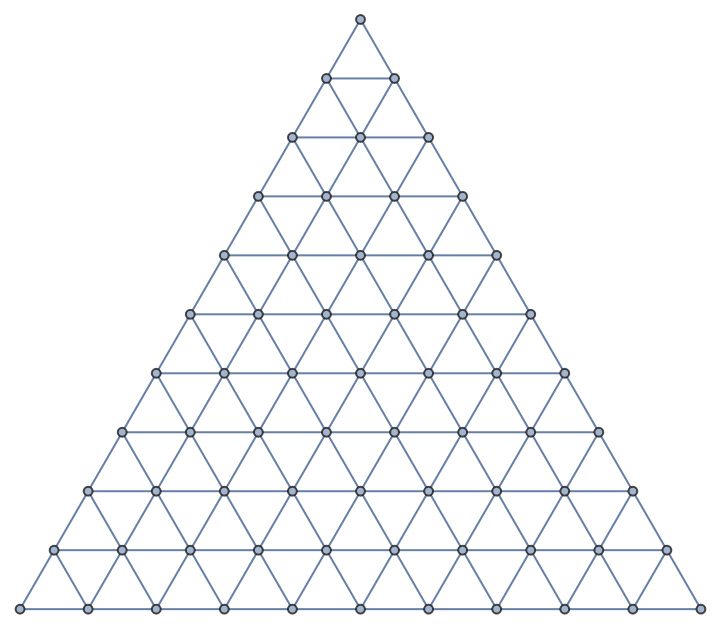Order 2 has 2 triangles and 3 vertices on each side:

 In:=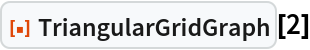Out=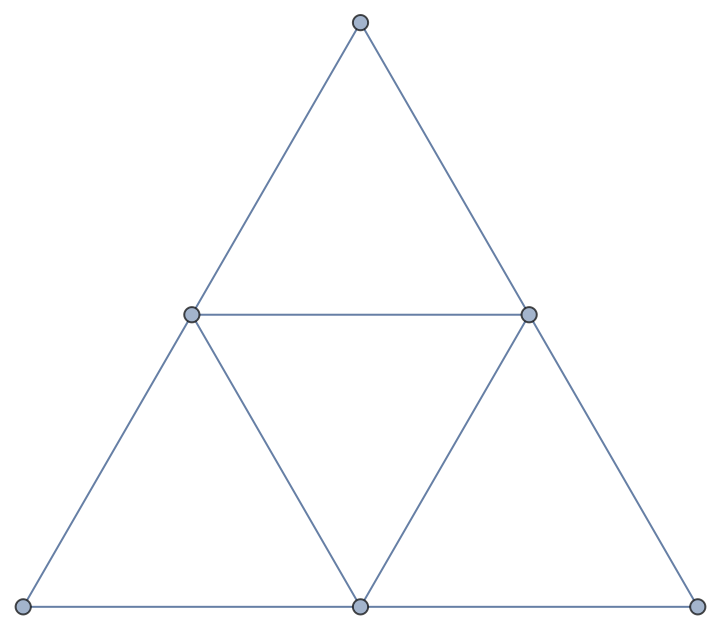### Properties and Relations (2)

Compare grid graphs corresponding to different Euclidean tilings:

 In:=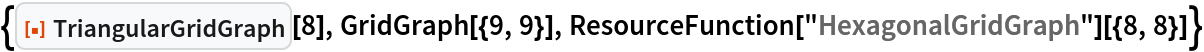Out=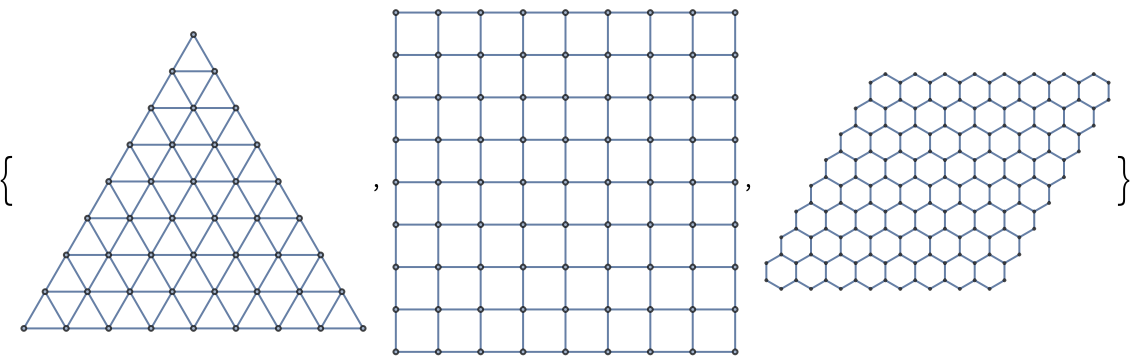Compare a triangular grid graph and a graph obtained from the Sierpinski triangle:

 In:=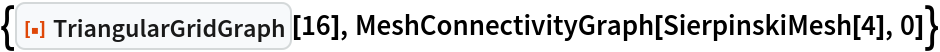Out=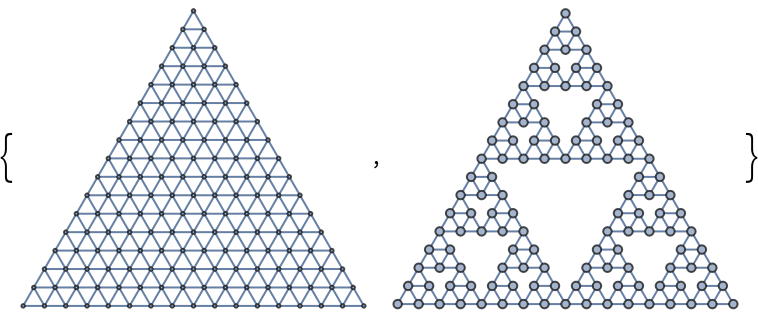## Publisher

Wolfram Summer Camp

## Version History

• 1.0.0 – 01 August 2022